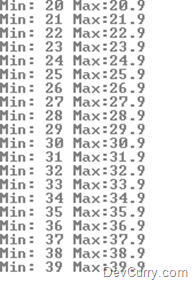# Divide Sequence into Groups and Query using LINQ

 Tweet

Yesterday, I had blogged about Querying a Sequence using LINQ. Now let us say if this sequence was to be divided into smaller sequences/batches and then queried upon, here’s how we would do it using LINQ

We will divide the sequence we generated into a group of 10’s and find the minimum and maximum value in each group. Use the following code:

`static void Main(string[] args){    var sequence = Enumerable.Range(200, 200).Select(x => x / 10f);    var grps = from x in sequence.Select((i, j) => new { i, Grp = j / 10 })                group x.i by x.Grp into y                select new { Min = y.Min(), Max = y.Max() };    foreach(var grp in grps)    Console.WriteLine("Min: " + grp.Min + " Max:" + grp.Max);    Console.ReadLine();}`

The query shown above first projects each element of a sequence into a new form and groups by 10. The results are shaped into an enumerable collection of anonymous objects with a property Min and Max. These values are then printed on the console, as shown below:

OUTPUT